# NCERT Exemplar: Magnetic Effects of Electric Current Notes | Study Science Class 10 - Class 10

## Class 10: NCERT Exemplar: Magnetic Effects of Electric Current Notes | Study Science Class 10 - Class 10

The document NCERT Exemplar: Magnetic Effects of Electric Current Notes | Study Science Class 10 - Class 10 is a part of the Class 10 Course Science Class 10.
All you need of Class 10 at this link: Class 10

Q 1. What is the role of the two conducting stationary brushes in a simple electric motor?
Ans: The two stationary conducting brushes make the contact in between the two halves of the split rings, and the source battery. Current in the coil enters from the source battery through one conducting brush and flows back to the battery through another brush.

Q 2. A magnetic compass needle is placed in the plane of paper near points A as shown in figure. In which plane should a straight current carrying conductor be placed so that it passes through A and there is no change in the deflection of the compass? Under what condition is the deflection maximum and why?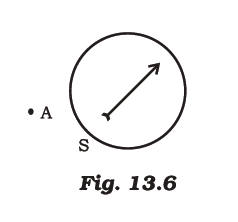Ans: In the given situation, a straight current carrying conductor should be placed in the plane of paper. The field produced by it is perpendicular to the plane of paper and parallel to the vertical axis of compass needle. As a result there will be no change in the deflection of compass needle.
The deflection is maximum when the conductor through the point A is perpendicular to the plane of paper so that field due to it is parallel to the plane of paper.

Q 3. AB is a current carrying conductor in the plane of the paper as shown in figure. What are the directions of magnetic fields produced by it at points P and Q? Given r> r2, where will the strength of the magnetic field be larger?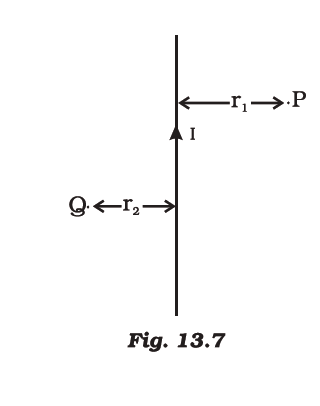Ans: Apply right-hand thumb rule, the direction of magnetic field produced by a current carrying conductor at Point
P - into the plane of paper Point
Q - out of the plane of paper.
As magnetic field is inversely proportional to distance, the field at Q will be larger than the field at P (r1 > r2).

Q 4. It is established that an electric current through a metallic conductor produces a magnetic field around it. Is there a similar magnetic field produced around a thin beam of moving (i) alpha particles, (ii) neutrons?

Ans: (i) Yes, similar magnetic field produced around a thin beam of moving alpha-particle, α-particle being positively charged constitutes a current in the direction of beam motion.
(ii) No, as neutron being electrically neutral and does not constitute a current.

Q 5. Meena draws magnetic field lines of field close to the axis o f a current carrying circular loop. As she moves away from the centre of the circular loop, she observes that the lines keep on diverging. How will you explain her observation?
Ans: Strength of magnetic field at a point decreases as the distance increases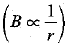Therefore as she moves away from the centre of circular coil, magnetic field decreases. This is indicated by the decrease in degree of closeness of the field lines and appears diverging in nature.

Q 6. Name four appliances wherein an electric motor, a rotating device that converts electrical energy to mechanical energy, is used as an important component. In what respect motors are different from generators?
Ans:
Electric fans, mixers, washing machines, computer drives, are the examples wherein an electric motor, a rotating device that converts electrical energy to mechanical energy, is used as an important component.

Motors convert electrical energy into mechanical energy whereas generators convert mechanical energy into electrical energy.

Q 7. Explain the phenomenon of electromagnetic induction. Describe an experiment to show that a current is set up in a closed loop when an external magnetic field passing through the loop increases or decreases.
Ans: Electromagnetic Induction: The process by which a changing magnetic field in a conductor induces a current in another conductor placed near it, is called electromagnetic induction.
Aim: To show that a current set up in a closed loop due to magnetic field passing through it varies.
Apparatus required: Two insulted copper coils having different number of turns, battery, plug key, rheostat, galvanometer, connecting wires and nonconducting cylindrical roll.
Procedure:
1. Insert the copper coils over a non-conducting cylinder.
2. Connect the free ends of coil A called primary coil having large number of turns in series with battery and a plug key.
3. Connect the coil B called secondary coil with a galvanometer as shown.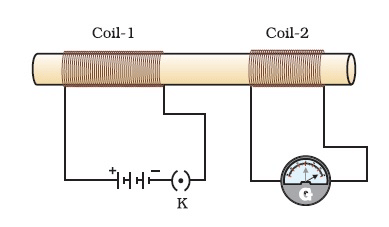Deflection is observed in the Galvanometer when Current flowing through coil-1 is changed.4. Plug the key and observe, if there is any momentary deflection in the galvanometer.
5. Disconnect the primary coil, i.e. coil A from the battery by unplugging the key. Again observe the needle movement.
6. Increase or decrease the current in the primary coil by varying the rheostat terminal and observe the degree of needle deflection in the galvanometer.
Observation:
1. When plugging the key, the needle of galvanometer deflects in one direction and quickly returns to zero.
2. When the current is switched off, needle momentarily moves in the opposite direction as observed in previous case.
3. Increasing/decreasing the current in primary coil leads to variation in the deflection of needle.
Conclusion:
1. Potential difference is induced in the secondary coil due to changes in magnetic field associated with it. It is due to change in the electric current through the primary coil.
2. Due to induced potential difference, a current is set up in the secondary coil which causes deflection in the galvanometer.
Thus, a current is set up in a closed loop when an external magnetic field passing through the loop increases (at the time of starting) or decreases (at the time of stopping).

Q 8. Draw an appropriate schematic diagram showing common domestic circuits and discuss the importance of fuse. Why is it that a burnt out fuse should be replaced by another fuse of identical rating?
Ans:

• A schematic diagram for common domestic circuit: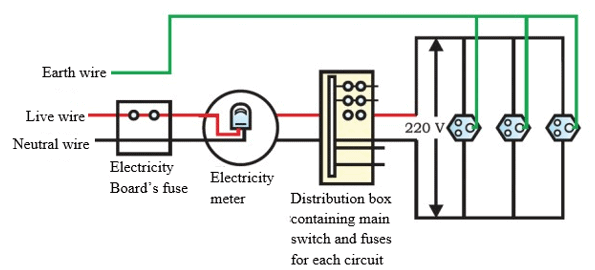• Importance of fuse: It is the most important safety device, used for protecting the circuit from short circuiting or overloading.
• Burnt fuse in the live wire acts as an open circuit. So, to maintain the flow of current in the circuit and to prevent the circuit from damage due to some fault, a burnt out fuse should be replaced by another fuse of identical rating.
The document NCERT Exemplar: Magnetic Effects of Electric Current Notes | Study Science Class 10 - Class 10 is a part of the Class 10 Course Science Class 10.
All you need of Class 10 at this link: Class 10Use Code STAYHOME200 and get INR 200 additional OFF

## Science Class 10

107 videos|325 docs|162 tests

Track your progress, build streaks, highlight & save important lessons and more!

,

,

,

,

,

,

,

,

,

,

,

,

,

,

,

,

,

,

,

,

,

;